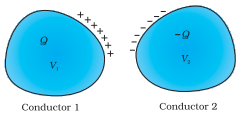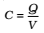# Capacitor and Capacitance – Formula, Uses, Factors Affecting

0
93

## What Is a Capacitor?

A capacitor is a two-terminal electrical device that can store energy in the form of an electric charge. It consists of two electrical conductors that are separated by a distance.  The space between the conductors may be filled by vacuum or with an insulating material known as a dielectric. The ability of the capacitor to store charges is known as capacitance.

Capacitors store energy by holding apart pairs of opposite charges. The simplest design for a capacitor is a parallel plate, which consists of two metal plates with a gap between them. But, different types of capacitors are manufactured in many forms, styles, lengths, girths, and materials.

## Capacitor and Capacitance

• A capacitor (formerly known as condenser) is a device that can store electronic charge and energy.
• All capacitors consist of a combination of two conductors separated by an insulator.
• The insulator is called dielectric which could be oil, air or paper and much more such materials are there which can act as a dielectric medium between conducting plates of a capacitor.
• The conductors have charges; say Q1 and Q2, and potentials V1 and V2. Usually, in practice, the two conductors have charges Q and – Q, with potential difference V = V1 – V2 between them.A system of two conductors separated by an insulator forms a capacitor.

The conductors may be so charged by connecting them to the two terminals of a battery. Q is called the charge of the capacitor, though this, in fact, is the charge on one of the conductors – the total charge of the capacitor is zero.

The electric field in the region between the conductors is proportional to the charge Q. That is, if the charge on the capacitor is, say doubled, the electric field will also be doubled at every point.

The potential difference V is the work done per unit positive charge in taking a small test charge from the conductor 2 to 1 against the field.Consequently, V is also proportional to Q, and the ratio Q/V is a constant C. The constant C is called the capacitance of the capacitor. C is independent of Q or V, as stated above.

• The SI unit of capacitance is 1farad (=1coulomb volt-1) or 1F = 1CV–1. A capacitor with fixed capacitance is symbolically shown as• The maximum electric field that a dielectric medium can withstand without break-down (of its insulating property) is called its dielectric strength; for air, it is about 3 × 106 Vm–1.